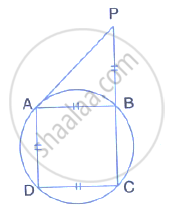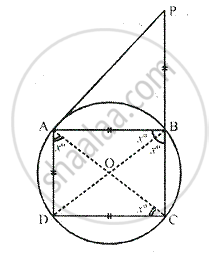Share

# In the Given Figure, Ab = Ad = Dc = Pb and ∠Dbc = X°. Determine, in Terms of X : (I) ∠Abd, (Ii) ∠Apb Hence Or Otherwise, Prove that Ap is Parallel to Db. - Mathematics

Course

#### Question

In the given figure, AB = AD = DC = PB and ∠DBC = x°. determine, in terms of x : (i) ∠ABD, (ii) ∠APB
Hence or otherwise, prove that AP is parallel to DB.#### SolutionGiven – In the figure, AB = AD = DC = PB and DBC = X
Join AC and BD
To find : the measure of ∠ABD and ∠APB

Proof : ∠DAC = ∠DBC = X

[angels in the same segment]

But ∠DCA = ∠DAC = X    [ ∵ AD = DC ]

Also, we have, ∠ABD = ∠DAC  [angles in the same segment]

In ∆ABP, ext ∠ABC = ∠BAP + ∠APB

But, ∠BAP = ∠APB     [ ∵ AB = BP]

2 × X = ∠APB+ ∠APB = 2∠APB
∴ 2∠APB = 2X
⇒  ∠APB = X
∴ ∠APB = ∠DBC = X ,
But these are corresponding angles
∴ AP || DB

Is there an error in this question or solution?